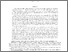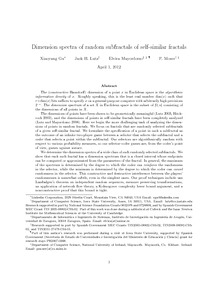# Dimension spectra of random subfractals of self-similar fractals

Gu, Xiaoyang and Lutzy, Jack H. and Mayordomo, Elvira and Moser, Philippe (2014) Dimension spectra of random subfractals of self-similar fractals. Annals of Pure and Applied Logic, 165 (11). pp. 1707-1726. ISSN 0168-0072Previewmore...Add this article to your Mendeley library

## Abstract

The (constructive Hausdorff) dimension of a point x in Euclidean space is the algorithmic information density of x. Roughly speaking, this is the least real number dim(x) such that r x dim(x) bits suffices to specify x on a general-purpose computer with arbitrarily high precisions 2-r. The dimension spectrum of a set X in Euclidean space is the subset of [0,n] consisting of the dimensions of all points in X. The dimensions of points have been shown to be geometrically meaningful (Lutz 2003, Hitchcock 2003), and the dimensions of points in self-similar fractals have been completely analyzed (Lutz and Mayordomo 2008). Here we begin the more challenging task of analyzing the dimensions of points in random fractals. We focus on fractals that are randomly selected subfractals of a given self-similar fractal. We formulate the specification of a point in such a subfractal as the outcome of an infinite two-player game between a selector that selects the subfractal and a coder that selects a point within the subfractal. Our selectors are algorithmically random with respect to various probability measures, so our selector-coder games are, from the coder's point of view, games against nature. We determine the dimension spectra of a wide class of such randomly selected subfractals. We show that each such fractal has a dimension spectrum that is a closed interval whose endpoints can be computed or approximated from the parameters of the fractal. In general, the maximum of the spectrum is determined by the degree to which the coder can reinforce the randomness in the selector, while the minimum is determined by the degree to which the coder can cancel randomness in the selector. This constructive and destructive interference between the players' randomnesses is somewhat subtle, even in the simplest cases. Our proof techniques include van Lambalgen's theorem on independent random sequences, measure preserving transformations, an application of network flow theory, a Kolmogorov complexity lower bound argument, and a nonconstructive proof that this bound is tight.

Item Type: Article This is the preprint version of the published article, which is available at DOI: 10.1016/j.apal.2014.07.001 Effective dimension; Random self-similar fractal; Dimension spectra; Faculty of Science and Engineering > Computer Science 6524 https://doi.org/10.1016/j.apal.2014.07.001 Philippe Moser 04 Nov 2015 14:47 Annals of Pure and Applied Logic Elsevier Yes

### Repository Staff Only(login required)Item control page